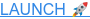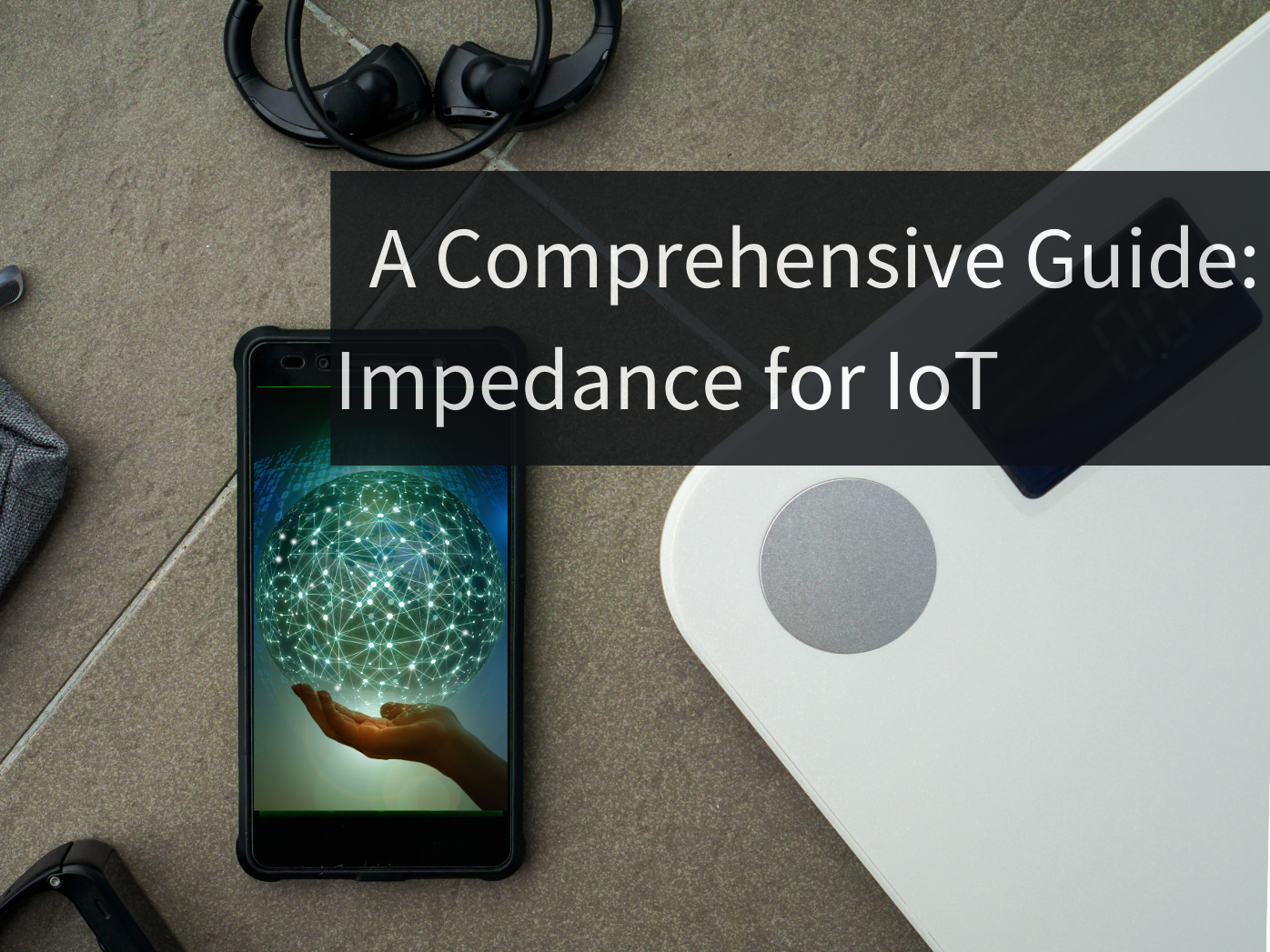# A Comprehensive Guide: Impedance for IoT

Impedance, often viewed as a complex concept, lies at the heart of PCB design, especially in high-speed applications. This guide aims to unravel its intricacies, demonstrating its critical role in ensuring signal integrity and reliable performance in modern electronics.## What is Impedance?

Based on the Oxford English Dictionary, electrical impedance is defined as:

The total opposition presented by a circuit or device to the flow of alternating current, comprising resistance, inductance, and capacitance.

In other words, impedance is a measure of how difficult it is for alternating current (AC) to flow through a circuit.

## Impedance vs Resistance

Impedance acts in a similar way to resistance in DC circuits. However, in AC circuits, resistance serves as a component of impedance. Let's further dive into the difference between the two before getting more familiar with impedance.

 Impedance (Z） Resistance (R) Property Vector, made up of Resistance (R) and Reactance (X).  Z = R + jX Scalar. Units Measured in ohms (Ω) Measured in ohms (Ω). Circuit type AC circuit DC circuit Phase relationship NO phase shift between voltage and current Reactance might introduce phase shift in voltage and current Power dissipation Real power dissipation (P = V^2 / R) and heating effects. Both real and reactive power dissipation in AC circuits
Table 1 Resistance vs Impedance

## Reactance, Capacitance, Inductance

Reactance, the vector component of impedance, is made up of capacitance and inductance.

Hence, the equation for impedance can be formulated to be:

Impedance (Z) = Reactance (X) j + Resistance

Impedance (Z) = [Capacitance (C) + Inductance (L)] j + Resistance

Where indicates an imaginary value.Figure 1 Voltage and Current phases with resistance, inductance, and capacitance.

### Reactance

Reactance is the component of impedance that accounts for the phase shift in AC circuits.

### Inductance

Inductance is the property of a material or component to store energy in a magnetic field when current flows through it. It's measured in Henrys (H).

Inductance contributes to inductive reactance, which is measured by

Z=jωL

where Z is the reactance, ω is the frequency in rad/s, and L is the inductance.

### Capacitance

Capacitance is the ability of a material or component to store electrical energy in an electric field. It's measured in Farads (F).

Capacitance contributes to capacitive reactance, which is measured by

Z = -j*(1/ωC)

where Z is the reactance, ω is the frequency in rad/s, and C is the capacitance.

## Impedance Importance for IoT Applications

Optimizing impedance is always an iterative process, especially during the process of designing PCBs. IoT PCBs, most importantly, should have optimized impedance design to function properly. This includes several factors, such as:

• Signal Integrity: Proper impedance matching ensures signals are transmitted accurately, preventing distortion or loss of data. This is critical for reliable communication in IoT devices.

• Wireless Connectivity: Impedance control is crucial for optimizing antenna performance. It ensures that signals are efficiently transmitted and received, enhancing wireless connectivity in IoT applications.

• Reducing Interference: Consistent impedance values help minimize electromagnetic interference (EMI) and cross-talk between traces. This is vital in densely populated IoT PCBs to prevent signal degradation.

• Power Efficiency: Impedance control helps in efficient power distribution across the PCB. This is crucial for battery-powered IoT devices, as it minimizes energy wastage and prolongs battery life.

• RF Performance: In IoT devices that use wireless communication protocols, such as Wi-Fi or Bluetooth, maintaining proper impedance is essential for maximizing RF signal strength and range.

• Sensitivity to Environmental Factors: IoT devices often operate in varied environments. Impedance control helps in ensuring consistent performance, even in conditions with temperature fluctuations or exposure to moisture.

## Conclusion

Impedance is a measure of electrical resistance in AC circuits that takes into consideration both resistance and reactance. Reactance itself is also made up of two other components, capacitive and inductive reactance.

In the IoT realm, Impedance is essential as it ensures the authenticity of signals passing via IoT devices and enables consistent connectivity. In addition, strict impedance management should be implemented to reduce electromagnetic interference and enhance signal integrity. This is especially important in wireless communication-dependent IoT devices, where antenna performance is heavily influenced by impedance tuning. As a result, impedance is an important issue when designing IoT devices that work smoothly and efficiently.